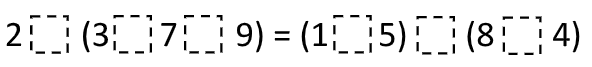# Balanced Equation

Directions: Use the operation symbols (+, -, x, and ÷) to make the equation true. Operations may be used more than once.### Hint

What is a value for the left side of the equation? What is a value for the right side of the equation?

There are many possibilities, here is one
2 x (3 x 7 + 9) = (1 x 5) x (8 +4)

Source: Joshua Nelson

## Add Fractions with Decimal Sums

Directions: Using the digits 1 to 9 at most one time each, place a digit …

1.My class got 2 x (3 + 7 + 9) = (1 + 5) + (8 x 4)

2.2+(3×7=9)=(1-5)/(8×4)
2x(3/7-9)=(1+5)x(8/4)
2-(3x7x9)=(1-5)-(8/4)
2/(3-7/9)=(1×5)98-4)
2x(3+7+9)=(1-5)(8×4)

•2×(3×7+9)=(1×5)×(8+4)

•you helped me alot

•We got
2X(3×7-9) = (1+5×8-4)

•Jennifer Cecchine

Both sides need to be equal. In most of your examples, you have either a negative solution on only one side, or you have a fractional answer on only one side.

2+(3×7=9)=(1-5)/(8×4)
2 + (21-9) = -4 ÷ 32 (I assumed you meant -9, not =9
2 +12 = -⅛ (or -0.125)
14 ≠ -⅛

2x(3/7-9)=(1+5)x(8/4)
2 x (3 ÷ 7 – 9) = (1 + 5) x (8 ÷ 4)
2 x (3/7 – 9) = 6 x 2
2 x -8 4/7 = 12
-17 1/7 ≠ 12

2-(3x7x9)=(1-5)-(8/4)
2 – (21 x 9) = -4 – 2
2 – 189 = -6
-187 ≠ -6

2/(3-7/9)=(1×5)98-4)
2 ÷ (3 – 7/9) = 5 x 4 (I assumed you meant x, not 9)
2 ÷ 2 2/9 = 20
9/10 ≠ 20

2x(3+7+9)=(1-5)(8×4)
2 x 19 = -4 x 32
38 ≠ -128

You were almost there. On your last equation, can you rework the second expression to equal the value of the first expression?

3.2 x (3 + 7 + 9)=(1 + 5) + (8 x 4)

4.I got 2 times (3 times 7 + 9)=( 1 times 5)times ( 8+4)

5.2- (3X7-9)=(1X5)X(8+4)

6.2x (3+7+9)=(1-5)(8×4)

7.2x (3+7+9)=(1-5)(8×4)

8.2 x (3 x 7 + 9) = (1 x 5) x (8 +4)

•2x(3×7+9)=(1×5)x(8+4)

9.Garrison Phillips

2 x (3 x 7 + 9) = (1 x 5) x (8 + 4)

10.2 X (3×7+9)=(1×5)x(8+4)

•I kinda do but don’t you put what you think goes were and you pute them there

11.2 x (3 x 7 + 9) = (1 x 5) x (8 +4) Is the one that I got.

12.i don understand the equation

•2-(3+7-9)=(1×5)-(8-4)

13.I got 2×(3×7+9)=(1-5)×(8+1)

14.2 x (3 x 7 + 9) = (1 x 5) x (8 +4)

15.I got 2 +(3-7+9)=(1×5)+(8/4) Answer 7.

16.17.2x(3×7+9)=(1×5)x(8+\$

•2x(3×7+9)=(1×5)x(8=4

18.2x(3=7=9)=(1×5)x(8=4)

19.2x(3×7+9) = (1×5)x(8+4)

20.2 x (3 x 7 + 9) = (1 x 5) x (8 +4)

21.2x(3×7+9)=(1×5)x(8+4)

•Wow Trevor this was actually helpful.

22.2x(3-7+9)=(1+5)-(8×4)

•OMG. Thank you so much. All the other ones are wrong after I tried doing it. You are right. Thank you Thank you Thank you!

23.2 + ( 3 + 7 – 9 ) = ( 1 x 5 ) – ( 8 / 4 )

24.2 x [3+7+9]=[1+5+4 x 8]

25.2 x (3 x 7 + 9) = (1 x 5) x (8 +4)

26.2x(3+7+9)=(1+5)+(8×4)

27.2x(3+7+9)=(1+5)+8×4}

28.We got
2x(3×7-9) = (1+5)x(8-4)

29.2×(3x7x9)=(1×5)x(8×4)

30.2x(3×7+9)=(1×5)x(8+4)

31.I got 2-(3+7-9)=(1/5)-(8-4)